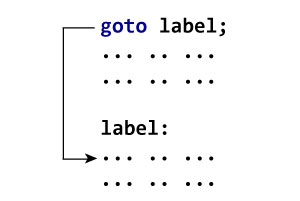C goto Statement

# C goto Statement

#### In this tutorial, you will learn to create the goto statement in C programming. Also, you will learn when to use a goto statement and when not to use it.

The `goto` statement allows us to transfer control of the program to the specified label.

### Syntax of goto Statement

``````goto label;
... .. ...
... .. ...
label:
statement;
``````

The label is an identifier. When the `goto` statement is encountered, the control of the program jumps to `label:` and starts executing the code.### Example: goto Statement

``````// Program to calculate the sum and average of positive numbers
// If the user enters a negative number, the sum and average are displayed.

#include <stdio.h>

int main() {

const int maxInput = 100;
int i;
double number, average, sum = 0.0;

for (i = 1; i <= maxInput; ++i) {
printf("%d. Enter a number: ", i);
scanf("%lf", &number);

// go to jump if the user enters a negative number
if (number < 0.0) {
goto jump;
}
sum += number;
}

jump:
average = sum / (i - 1);
printf("Sum = %.2f\n", sum);
printf("Average = %.2f", average);

return 0;
}``````

Output

```1. Enter a number: 3
2. Enter a number: 4.3
3. Enter a number: 9.3
4. Enter a number: -2.9
Sum = 16.60
Average = 5.53```

### Reasons to avoid goto

The use of `goto` statement may lead to code that is buggy and hard to follow. For example,

``````one:
for (i = 0; i < number; ++i)
{
test += i;
goto two;
}
two:
if (test > 5) {
goto three;
}
... .. ...
``````

Also, the `goto` statement allows you to do bad stuff such as jump out of the scope.

That being said, `goto` can be useful sometimes. For example: to break from nested loops.

### Should you use goto?

If you think the use of `goto` statement simplifies your program, you can use it. That being said, `goto` is rarely useful and you can create any C program without using `goto` altogether.

Here's a quote from Bjarne Stroustrup, creator of C++, "The fact that 'goto' can do anything is exactly why we don't use it."